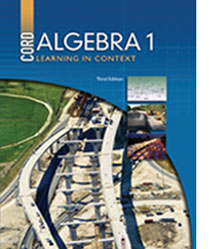# Algebra 1 - 3rd Edition

## Chapter 7: Statistics

Some links are repeated for use with more than one lesson.

### These sites apply to the entire chapter.

http://www.freemathhelp.com/Lessons/Algebra_2_Probability_and_Statistics_BB.htm
This page contains a video tutorial with notes covering an introduction to probability and statistics.

http://www.robertniles.com/stats/
This site defines the different components of statistics.

### 7.1 Measures of Central Tendency

This page contains word problems that practice the measures of central tendency.

### 7.3 Scatter Plots, Correlation, and Lines of Best Fit

http://staff.argyll.epsb.ca/jreed/math9/strand4/scatterPlot.htm
This site defines scatter plots and lines of best fit and has interactive graph applets on which to plot points.

### 7.4 Stem-and-Leaf Plots

http://www.mathsisfun.com/definitions/stem-and-leaf-plot.html
This site contains a quick guide to using a stem-and-leaf plot.

http://regentsprep.org/regents/math/stemleaf.htm
NEW This page has an explanation of stem-and-leaf plots with examples of how to construct them and an explanation of the special case ones.

### 7.5 Quartiles and Box-and-Whisker Plots

http://www.purplemath.com/modules/boxwhisk.htm
This site explains quartiles and box-and-whisker plots through text and graphs.